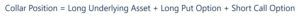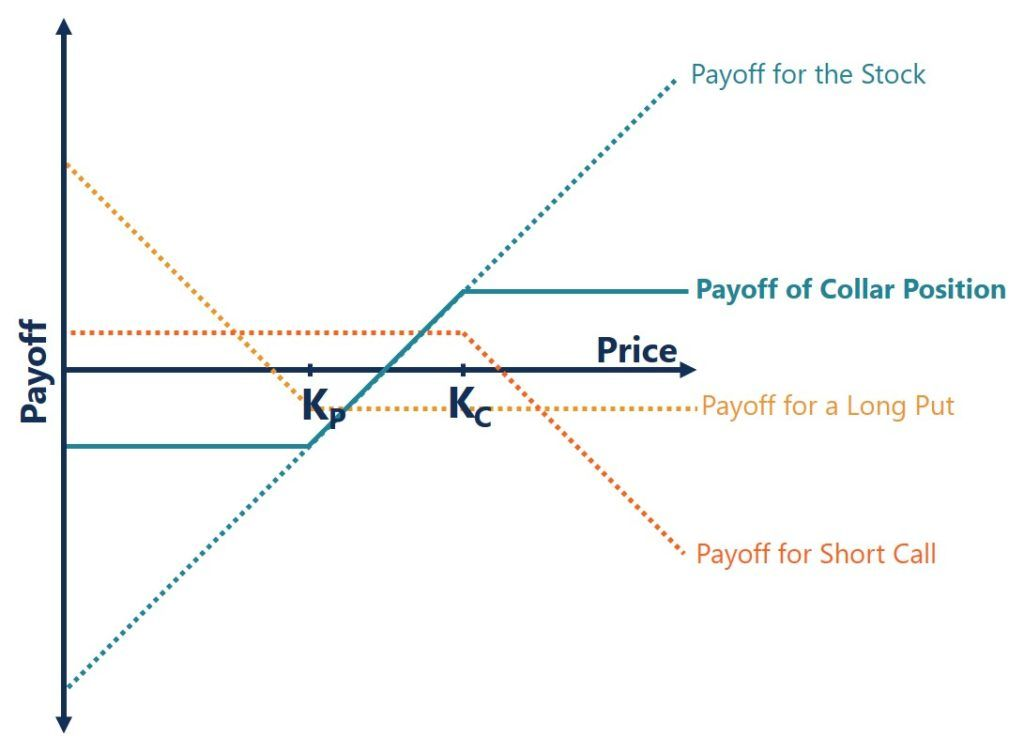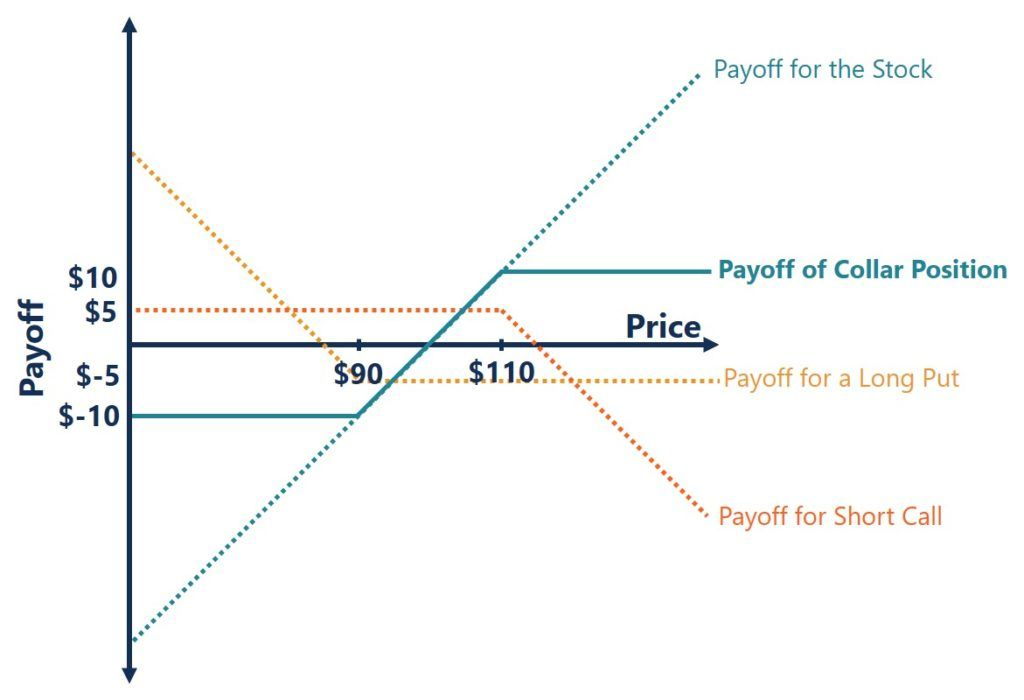# Collar Option Strategy

An option strategy that limits both upside and downside

## What is a Collar Option Strategy?

A collar option strategy, also referred to as a hedge wrapper or simply collar, is an option strategy employed to reduce both positive and negative returns of an underlying asset. It limits the return of the portfolio to a specified range and can hedge a position against potential volatility of the underlying asset. A collar position is created through the usage of a protective put and covered call option. More specifically, it is created by holding an underlying stock, buying an out of the money put option, and selling an out of the money call option.### Quick Summary

• A collar option strategy is an option strategy that limits both gains and losses.
• A collar position is created by holding an underlying stock, buying an out of the money put option, and selling an out of the money call option.
• Collars may be used when investors want to hedge a long position in the underlying asset from short-term downside risk.

### Creating a Collar Position### Interpreting the Collar Option Strategy

The collar option strategy will limit both upside and downside. The collar position involves a long position on an underlying stock, a long position on the out of the money put option, and a short position on the out of the money call option.

By taking a long position in the underlying stock, as the price increases, the investor will profit. As the price decreases, the investor will experience a loss. Holding a long position on an out of the money put option, as the price of the underlying stock decreases, the put option value increases. We see here that the downside of a falling stock price is neutralized by the put option.

The investor will also take a short position on an out of the money call option. If the price of the underlying stock increases, the call option will be exercised by the buyer. Therefore, as the seller, you will experience a loss as the underlying asset increases in price. This potential loss neutralizes the upside of holding the stock.

The value of the underlying asset between the strike price of the put option and call option is the value of the portfolio that moves. The loss experienced by the call option above the call strike price will cancel with gains from the stock appreciating, therefore the payoff will be flat here. The gains experienced by the put option below the strike price will cancel with the loss from the depreciating stock price. The payoff will also be flat here. Below we can see what the payoff diagram of a collar would look like.

### Collar Option Payoff Diagram

The payoff of a collar can be understood through the use of a payoff diagram. By plotting the payoff for the underlying asset, long put option, and short call option we can see what the collar position payoff would be:In the chart above, we see that below the put strike price (Kp) and above the call strike price (Kc), the payoff is flat. The potential upside and downside of the portfolio are limited. It is only between the strike prices that we see the payoff movement of a collar position.

### Uses of the Collar Option Strategy

The collar option strategy is most often used as a flexible hedging option. If an investor holds a long position on a stock, they can construct a collar position to protect against large losses. It is through the usage of the protective put option that will gain when the underlying asset falls in price. The covered call option is sold which can be used to pay for the put option and it will still allow potential upside from an appreciation in the underlying asset, up to the call’s strike price. When the entire cost of the put option is covered by selling the call option, this is referred to as the zero-cost collar.

If a stock has strong long-term potential, but in the short-term has high down-side risk then a collar can be considered. Investors will also consider a collar strategy if a stock they are long in has recently appreciated significantly. To protect these unrealized gains a collar may be used. The use of a collar strategy is also used in mergers and acquisitions. In a stock deal, a collar can be used to ensure that a potential depreciation of the acquirers stock does not lead to a situation where they must pay much more in diluted shares.

### Collar Option Strategy – Worked Example

Let us now look at an example that involves creating a collar. Say you are holding a long position on an asset that has just recently appreciated to a price of \$100. You are unsure about the price stability in the near-term future and want to utilize a collar strategy. You buy a put option with a strike price of \$90 at a premium of \$5. You also sell a call option for \$5 with a strike price of \$110.

What is your payoff if the price of the asset falls to \$80?

• The call option you’ve sold will not be exercised by the buyer and you will end with a payoff of \$5.
• The put option you’ve bought for \$5 will be exercised with a strike price of \$90 meaning a payoff of \$5.
• The underlying asset will be worth \$80 meaning a loss of \$20.

The protective put option you’ve purchased reduced the losses experienced from a drop in the price of the underlying asset. In total your net loss will be: \$5 + \$5 – \$20 = -\$10.

Rather than experiencing the full loss of \$80 – \$100 = -\$20, you have ended with a net loss of only \$10.

What is your payoff if the price of the asset increases to \$105?

• The call option you’ve sold for \$5 will not be exercised since the underlying asset price is still below the strike price. You will end with a payoff of \$5.
• The put option you’ve bought will not be exercised since the underlying price is above the strike price. You will realize a net loss of \$5.
• The underlying will be worth \$105, meaning a net gain of \$5.

The out of the money call and put were both not exercised. Selling the call option covered the cost of buying the put option so the payoff and loss from the two transactions canceled each other out. In total, your net payoff will be: \$5 – \$5 + \$5 = \$5.

It is the same payoff from just holding the underlying asset: \$105 – \$100 = \$5

What is your payoff if the price of the asset increases to \$115?

• The call option you’ve sold for \$5 will be exercised at a strike price of \$110. The payoff will be \$0.
• The put option you’ve bought for \$5 will not be exercised. The loss from the transaction will be -\$5.
• The underlying asset will be worth \$115, meaning a gain of \$15,

In this scenario, you would end up with a net payoff of \$0 – \$5 + \$15 = \$10.

It is less than if you had just held the underlying asset: \$115 – \$100 = \$15. In this scenario, the collar is limiting the upside potential of the underlying asset.

In the scenarios above, the call option you’ve sold completely covers the cost of buying the put option. It is called a zero-cost collar. Below is an illustration of the collar position:Here we can see that the loss is capped if the price of the underlying asset falls below \$90. Similarly, if the price of the underlying asset rises above \$110, the payoff is also capped. To further illustrate this, let’s look at two more scenarios.

What would the payoff be if the asset dropped in price to \$0?

• The call option you’ve sold for \$5 would not be exercised and the payoff would be \$5.
• The put option you’ve bought for \$5 would be exercised at a strike price of \$90. The payoff would be \$90 – \$5 = \$85.
• The underlying asset’s fallen from \$100 to \$0, resulting in a loss of \$100.

The net payoff to you would be \$5 + \$85 – \$100 = -\$10.

It is the same payoff when the asset fell to a price of \$80. We see that the protective put is capping the losses experienced from a fall in the underlying asset.

What would the payoff be if the asset rose in price to \$200?

• The call option you’ve sold for \$5 would be exercised and the payoff would be \$5 – \$90 = -\$85.
• The put option you’ve bought for \$5 would not be exercised. The loss from this transaction would be -\$5.
• The underlying asset’s risen from \$100 to \$200, resulting in a gain of \$100.

The net payoff to you would be -\$85 – \$5 + \$100 = \$10.

It is the same payoff when the price increased to \$115. Here, we can see how the collar position limited the upside potential of the underlying asset.

### Additional Resources

Thank you for reading CFI’s resource on the collar option strategy. If you would like to learn about related concepts, check out CFI’s other resources below:

• Hedging
• Protective Put
• Synthetic Position
• Options Case Study – Long Call

### Corporate Finance Training

Advance your career in investment banking, private equity, FP&A, treasury, corporate development and other areas of corporate finance.

Enroll in CFI’s Finance Courses to take your career to the next level! Learn step-by-step from professional Wall Street instructors today.